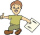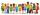# The ratio

The ratio of girls to boys in Mrs. White's class is 3:2.  If there are 12 boys, how many girls are in the class room?

g =  18

### Step-by-step explanation:Did you find an error or inaccuracy? Feel free to write us. Thank you!Tips to related online calculators
Need help to calculate sum, simplify or multiply fractions? Try our fraction calculator.
Check out our ratio calculator.

## Related math problems and questions:

• Boys and girls 8In a class of 35 boys and girls that has a ratio of 2:5, 5 boys are absent and 5 boys are present. How many students are present?
• Pupils 7There are 40 pupils in a certain class. 3/5 of the class are boys. How many are girls?
• FortyForty five of the 80 students were girls. What is the ratio of girls to boys?
• Ratio of countsThere are 15 boys and 13 girls in the class. What are the ratio of boys and girls?
• PupilsThere are 32 pupils in the classroom, and girls are two-thirds more than boys. a) How many percents are more girls than boys? Round the result to a whole percentage. b) How many boys are in the class? c) Find the ratio of boys and girls in the class. Writ
• GroupsIn the 6th class there are 60 girls and 72 boys. We want to divide them into groups so that the number of girls and boys is the same. How many groups can you create? How many girls will be in the group?
• A class IV.CIn a class 2/5 were boys. 30 were girls. How many more girls than boys are there?
• The classroomIn the seventh class the number of boys and girls are at a ratio of 4:5. Boys are 16. How many total students are in the classroom?
• There 12There are 42 students in the class and 2/3 of them are girls. How may girls are there in the class?
• Boys and girlsIf the ratio of male & female teachers is 2/5 & it is in proportion of the male & female students, how many are girls if there are 42 boys?
• Boys and girlsThere are 20 boys and 10 girls in the class. How many different dance pairs can we make of them?
• A class IV.AIn a class there are 15 girls and 30 boys.  What fraction of the class represents the boys?
• StudentsThere are 2/6 girls out of 1050 students at the school. Of these, 4/5 have another sibling. How many girls are in school? How many boys are in school? How many girls do not have a sibling?
• Boys and girlsThere are eight boys and nine girls in the class. There were six children on the trip from this class. What is the probability that left a) only boys b) just two boys
• Boys to girlsThe ratio of boys to girls at a party is 3:5. If six more boys arrived and four girls left the party, the ratio of boys to girls would be 5:6. How many people were at the party initially?
• Boys and glasses2/3 of the students in Sarah’s class are boys. Of the boys, 1/3  of them wear glasses. What fraction of the students wear glasses?
• Prospering studentsThere are girls and 30 boys in the class. Boys prospering 28, girls all. How many girls are in the class and how many boys are there, if all the prospering students are 95%?